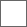### 【原创】诗词游戏联想对对碰，关键字为女明星的名字（第三场）（）（）（）（）（）（）（），（）（）（）叶（）（）（）。

（）（）青（）（），（）（）（）（）（）。

（）（）（）（）（）（）（），（）（）柴（）（）（）（）。

（）（）（）（）（）（）（），（）（）（）（）（）（）碧（）。

（）（）（）（）（）（）（），（）（）（）（）（）（）云。

（）（）（）（）（），（）孙（）（）（）。

（）（）（）佳（），（）（）（）（）（）。

（）（）（）雨（）（）（），（）（）（）（）（）（）（）。#### 扫二维码下载贴吧客户端